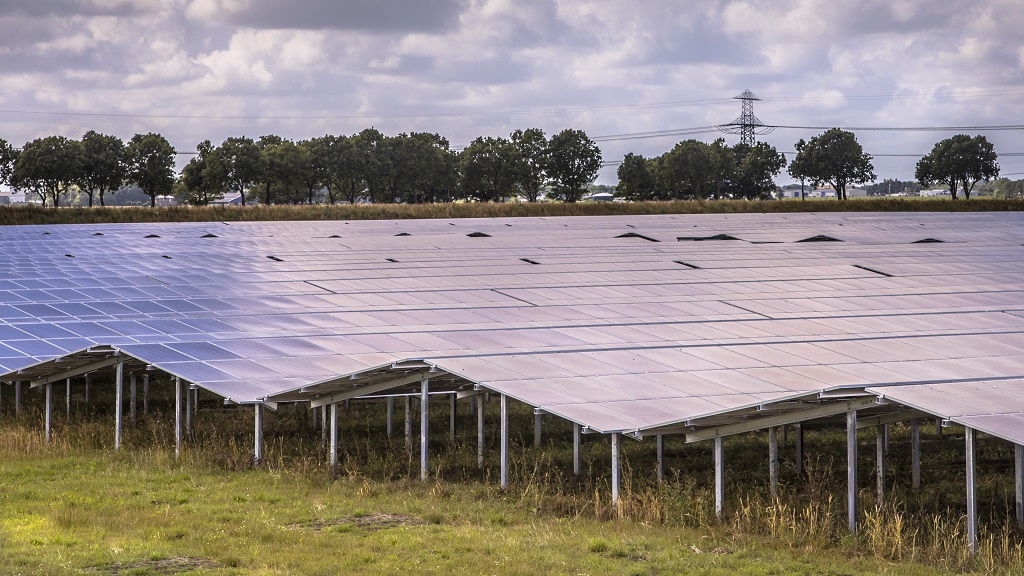## Alberta Commercial SolarThe average solar power system in Alberta will produce approximately 1276 kWh of energy per kW per year.

This yearly average decreases as you move north and west in the province and increases as you move south and east. For example:

• A 1kW solar system in Calgary would produce about 1,292 kWh/yr
• A 1kW solar system in Edmonton would produce about 1,246 kWh/yr
• A 1kW solar system in Red Deer would produce about 1,265 kWh/yr
• A 1kW solar system in Lethbridge would produce about 1,330 kWh/yr
• A 1kW solar system in Sherwood Park would produce 1,246 kWh/yr

Determining the size of system you need is as simple as knowing how much energy you use during the course of a year. Your monthly AB Power Bill will show your usage (in kWh) similar to the photo below:

You’ll need to figure out how much energy you use in a year by adding up the amount shown for 12 consecutive months. Note that taking one month and multiplying by 12 won’t work because energy use fluctuates depending on the season.

After you know how much energy you use, you can easily calculate the size of the solar power system that you’ll need by using the following equation:

Size of system needed (in kW) = yearly energy use (in kWh) / 1,276h

(where 1,276h equals the annual average equivalent of fullsunlight hours in Alberta)

So let’s pretend you added up your power bills and determined that you use 10,000kWh over the course of a year, you would then do the above calculation and determine that you need a 7.84kW solar panel system!

10,000kWh / 1,276h = 7.84kW